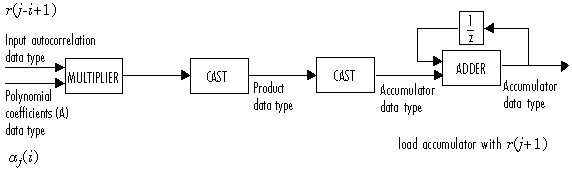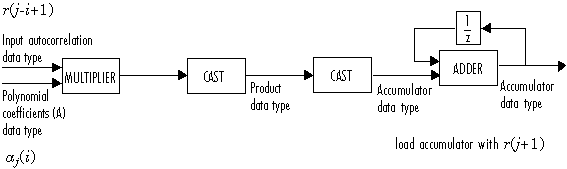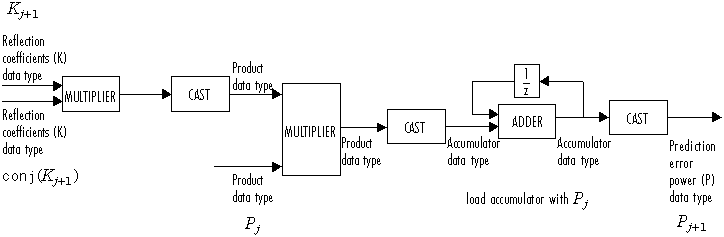# Levinson-Durbin

Solve linear system of equations using Levinson-Durbin recursion

## Library

Math Functions / Matrices and Linear Algebra / Linear System Solvers

`dspsolvers`

•## Description

The Levinson-Durbin block solves the nth-order system of linear equations

Ra = b

in the cases where:

• R is a Hermitian, positive-definite, Toeplitz matrix.

• b is identical to the first column of R shifted by one element and with the opposite sign.

The input to the block, ```r = ``````[r(1) r(2) ... r(n+1)]```, can be a vector or a matrix. If the input is a matrix, the block treats each column as an independent channel and solves it separately. Each channel of the input contains lags 0 through n of an autocorrelation sequence, which appear in the matrix R.

The block can output the polynomial coefficients, A, the reflection coefficients, K, and the prediction error power, P, in various combinations. The Output(s) parameter allows you to enable the A and K outputs by selecting one of the following settings:

• `A` — For each channel, port A outputs `A = ````[1 a(2) a(3) ... a(n+1)]```, the solution to the Levinson-Durbin equation. A has the same dimension as the input. You can also view the elements of each output channel as the coefficients of an nth-order autoregressive (AR) process.

• `K` — For each channel, port K outputs ```K = ``````[k(1) k(2) ... k(n)]```, which contains n reflection coefficients and has the same dimension as the input, less one element. A scalar input channel causes an error when you select `K`. You can use reflection coefficients to realize a lattice representation of the AR process described later in this page.

• `A and K` — The block outputs both representations at their respective ports. A scalar input channel causes an error when you select `A and K`.

Select the Output prediction error power (P) check box to output the prediction error power for each channel, P. For each channel, P represents the power of the output of an FIR filter with taps A and input autocorrelation described by r, where A represents a prediction error filter and r is the input to the block. In this case, A is a whitening filter. P has one element per input channel.

When you select the If the value of lag 0 is zero, A=[1 zeros], K=[zeros], P=0 check box (default), an input channel whose r`(1)` element is zero generates a zero-valued output. When you clear this check box, an input with r`(1)` = `0` generates `NaN`s in the output. In general, an input with r`(1)` = `0` is invalid because it does not construct a positive-definite matrix R. Often, however, blocks receive zero-valued inputs at the start of a simulation. The check box allows you to avoid propagating `NaN`s during this period.

### Applications

One application of the Levinson-Durbin formulation implemented by this block is in the Yule-Walker AR problem, which concerns modeling an unknown system as an autoregressive process. You would model such a process as the output of an all-pole IIR filter with white Gaussian noise input. In the Yule-Walker problem, the use of the signal's autocorrelation sequence to obtain an optimal estimate leads to an Ra = b equation of the type shown above, which is most efficiently solved by Levinson-Durbin recursion. In this case, the input to the block represents the autocorrelation sequence, with `r(1)` being the zero-lag value. The output at the block's A port then contains the coefficients of the autoregressive process that optimally models the system. The coefficients are ordered in descending powers of z, and the AR process is minimum phase. The prediction error, G, defines the gain for the unknown system, where $G=\sqrt{P}$:

The output at the block's K port contains the corresponding reflection coefficients, `[`k```(1) ```k```(2) ... ```k`(n)]`, for the lattice realization of this IIR filter. The Yule-Walker AR Estimator block implements this autocorrelation-based method for AR model estimation, while the Yule-Walker Method block extends the method to spectral estimation.

Another common application of the Levinson-Durbin algorithm is in linear predictive coding, which is concerned with finding the coefficients of a moving average (MA) process (or FIR filter) that predicts the next value of a signal from the current signal sample and a finite number of past samples. In this case, the input to the block represents the signal's autocorrelation sequence, with r`(1)` being the zero-lag value, and the output at the block's A port contains the coefficients of the predictive MA process (in descending powers of z).

These coefficients solve the following optimization problem:

`$\stackrel{\mathrm{min}}{\left\{{a}_{i}\right\}}$`
`$E\left[{|{x}_{n}-\sum _{i=1}^{N}{a}_{i}{x}_{n-i}|}^{2}\right]$`

Again, the output at the block's K port contains the corresponding reflection coefficients, `[k(1) k(2) ... k(n)]`, for the lattice realization of this FIR filter. The Autocorrelation LPC block in the Linear Prediction library implements this autocorrelation-based prediction method.

### Fixed-Point Data Types

The diagrams in this section show the data types used within the Levinson-Durbin block for fixed-point signals.

After initialization the block performs n updates. At the (j+1) update,

The following diagram displays the fixed-point data types used in this calculation:The block then updates the reflection coefficients K according to

The block then updates the prediction error power P according to

`${P}_{j+1}={P}_{j}-{P}_{j}×{K}_{j+1}×\text{conj}\left({K}_{j+1}\right)$`

The next diagram displays the fixed-point data types used in this calculation:The polynomial coefficients A are then updated according to

`${a}_{j+1}\left(i\right)={a}_{j}\left(i\right)+{K}_{j+1}×\text{conj}\left({\text{a}}_{\text{j}}\left(j-1+i\right)\right)$`

This diagram displays the fixed-point data types used in this calculation:## Algorithm

The algorithm requires O(n2) operations for each input channel. This implementation is therefore much more efficient for large n than standard Gaussian elimination, which requires O(n3) operations per channel.

## Parameters

Main Tab

Output(s)

Specify the solution representation of Ra = b to output: model coefficients (`A`), reflection coefficients (`K`), or both (`A and K`). When the input is a scalar or row vector, you must set this parameter to `A`.

Output prediction error power (P)

Select to output the prediction error at port P.

If the value of lag 0 is zero, A=[1 zeros], K=[zeros], P=0

When you select this check box and the first element of the input, `r(1)`, is zero, the block outputs the following vectors, as appropriate:

• `A = [1 zeros(1,n)]`

• `K = [zeros(1,n)]`

• `P = 0`

When you clear this check box, the block outputs a vector of `NaN`s for each channel whose `r(1)` element is zero.

Data Types Tab

Note

Floating-point inheritance takes precedence over the data type settings defined on this pane. When inputs are floating point, the block ignores these settings, and all internal data types are floating point.

Rounding mode

Specify the rounding mode for fixed-point operations as one of the following:

• `Floor`

• `Ceiling`

• `Convergent`

• `Nearest`

• `Round`

• `Simplest`

• `Zero`

For more details, see rounding mode.

Saturate on integer overflow

When you select this parameter, the block saturates the result of its fixed-point operation. When you clear this parameter, the block wraps the result of its fixed-point operation. For details on `saturate` and `wrap`, see overflow mode for fixed-point operations.

Product output

Specify the product output data type. See Fixed-Point Data Types and Multiplication Data Types for illustrations depicting the use of the product output data type in this block. You can set it to:

• A rule that inherits a data type, for example, `Inherit: Same as input`

• An expression that evaluates to a valid data type, for example, `fixdt(1,16,0)`

Click the buttonto display the Data Type Assistant, which helps you set the Product output parameter.

Accumulator

Specify the accumulator data type. See Fixed-Point Data Types for illustrations depicting the use of the accumulator data type in this block. You can set it to:

• A rule that inherits a data type, for example, `Inherit: Same as input`

• A rule that inherits a data type, for example, ```Inherit: Same as product output```

• An expression that evaluates to a valid data type, for example, `fixdt(1,16,0)`

Click the buttonto display the Data Type Assistant, which helps you set the Accumulator parameter.

Polynomial coefficients (A)

Specify the polynomial coefficients (A) data type. See Fixed-Point Data Types for illustrations depicting the use of the A data type in this block. You can set it to an expression that evaluates to a valid data type, for example, `fixdt(1,16,15)`.

Click the buttonto display the Data Type Assistant, which helps you set the A parameter.

Reflection coefficients (K)

Specify the polynomial coefficients (A) data type. See Fixed-Point Data Types for illustrations depicting the use of the K data type in this block. You can set it to an expression that evaluates to a valid data type, for example, `fixdt(1,16,15)`.

Click the buttonto display the Data Type Assistant, which helps you set the K parameter.

Prediction error power (P)

Specify the prediction error power (P) data type. See Fixed-Point Data Types for illustrations depicting the use of the P data type in this block. You can set it to:

• A rule that inherits a data type, for example, `Inherit: Same as input`

• An expression that evaluates to a valid data type, for example, `fixdt(1,16,0)`

Click the buttonto display the Data Type Assistant, which helps you set the P parameter.

Minimum

Specify the minimum values that the polynomial coefficients, reflection coefficients, or prediction error power should have. The default value is `[]` (unspecified). Simulink® uses this value to perform:

Maximum

Specify the maximum values that the polynomial coefficients, reflection coefficients, or prediction error power should have. The default value is `[]` (unspecified). Simulink uses this value to perform:

Lock data type settings against changes by the fixed-point tools

Select this parameter to prevent the fixed-point tools from overriding the data types you specify on the block mask.

## References

Golub, G. H. and C. F. Van Loan. Sect. 4.7 in Matrix Computations. 3rd ed. Baltimore, MD: Johns Hopkins University Press, 1996.

Ljung, L. System Identification: Theory for the User. Englewood Cliffs, NJ: Prentice Hall, 1987. Pgs. 278–280.

Kay, Steven M. Modern Spectral Estimation: Theory and Application. Englewood Cliffs, NJ: Prentice Hall, 1988.

## Supported Data Types

• Double-precision floating point

• Single-precision floating point

• Fixed point (signed only)

• 8-, 16-, and 32-bit signed integers

## Version History

Introduced before R2006a